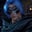Related Tags

java
communitycreator
python3

# How to solve a binary tree preorder traversal iterativelyGutha Vamsi Krishna

Traversing a tree means to visit all the nodes in a tree exactly once.

In a Pre-Order traversal, we visit all the nodes as follows:

1. Visit a node.
2. Visit the left child of the node.
3. Visit the right child of the node.

### Algorithm

The algorithm for a pre-order traversal of a binary tree is as follows:

• Initialize an array to store the traversed elements.
• Initialize an empty stack.
• Take the root element and push it into the stack.
• Use a while loop to perform the following steps until the stack is empty:
1. Remove the element at the top of the stack and add it to the array.
2. Check if the removed element has left or right children.
3. If the removed element does have children nodes, then push the right child into the stack first (if there is a right child), and then the left child (if a left child exists).
• When the stack becomes empty, the while loop will end, then the array that contains the elements in pre-order can be returned.

The right child is added to the stack before the left child so that we can pop the left element first, since the stack is a Last-in-First-Out (LIFO) data structure, and in a preorder traversal, the left child should be traversed before the right child.

The slides below illustrate the traversal process:

At first we initialize empty stack
1 of 11

### Implementation in Java

• Import the Java util package to use built-in data structures.
• The program starts from line 46.
• From lines 33 to 44, we create a node in a binary tree.
• In the main method, we use node objects to create a binary tree, as shown above.
• From lines 4 to 30, we define the solution class, which has the preorderTraversal method that returns a List.
• In the main method, in line 69, we create an object for the solution class. In line 72, we print out the list returned by the preorderTraversal method.

Refer to the comments provided in the code to understand what every line does.

import java.util.*;

//solution class
class Solution {
public List<Integer> preorderTraversal(TreeNode root) {
//initialize array list
List<Integer> preOrder = new ArrayList<>();

//if tree is empty then we return empty list
if(root == null) return preOrder;

//initialize stack
Stack<TreeNode> st = new Stack<TreeNode>();

//push root element to stack
st.push(root);

//loop runs till stack in not empty
while(!st.isEmpty()){
//pop the top element in stack
root = st.pop();
//check if left and right elements are present
if(root.right != null) st.push(root.right);
if(root.left != null) st.push(root.left);
}
return preOrder;
}
}

//class to create a new node in tree
class TreeNode {
int val;
TreeNode left;
TreeNode right;
TreeNode() {}
TreeNode(int val) { this.val = val; }
TreeNode(int val, TreeNode left, TreeNode right) {
this.val = val;
this.left = left;
this.right = right;
}
}

public class Main{
public static void main(String[] args){

//create instances of nodes
TreeNode node1 = new TreeNode(1);
TreeNode node2 = new TreeNode(2);
TreeNode node3 = new TreeNode(3);
TreeNode node4 = new TreeNode(4);
TreeNode node5 = new TreeNode(5);
TreeNode node6 = new TreeNode(6);
TreeNode node7 = new TreeNode(7);

//creating a binary tree as shown in above represention
node1.left = node2;
node1.right = node7;

node2.left = node3;
node2.right = node4;

node4.left = node5;
node4.right = node6;

//constructing solution object
Solution solution = new Solution();

//printing out the returned array list from solution
System.out.println(solution.preorderTraversal(node1));
}
}

### Implementation in Python3

• From lines 22 to 26, we create a node in a binary tree.
• From lines 29 to 45, we use node objects to create a binary tree, as shown above.
• From lines 2 to 19, where we defined a solution class, which has the preorderTraversal method. preorderTraversal returns a List that contains elements traversed in preorder fashion.
• In line 48, we create an object for the solution class and print out the list returned by the preorderTraversal method.

Refer to the comments provided in the code to understand what every line does.

#solution class
class Solution:
# pass root node as parameter
def preorderTraversal(self, root):
# initialize list
preorderList = []
# initialize stack with root element
stack = [root]
# while loop executes till stack is not empty
while stack:
#remove the top element from stack
node = stack.pop()
if node:
preorderList.append(node.val)
#add left and right elemens to stack
stack.append(node.right)
stack.append(node.left)
return preorderList

#class to create tree node
class TreeNode:
def __init__(self, val=0, left=None, right=None):
self.val = val
self.left = left
self.right = right

# create node instances
node1 = TreeNode(1);
node2 = TreeNode(2);
node3 = TreeNode(3);
node4 = TreeNode(4);
node5 = TreeNode(5);
node6 = TreeNode(6);
node7 = TreeNode(7);

# creates binary tree as shown in representation
node1.left = node2;
node1.right = node7;

node2.left = node3;
node2.right = node4;

node4.left = node5;
node4.right = node6;

# print the elements in preorder returned by solution
print(Solution().preorderTraversal(node1))

RELATED TAGS

java
communitycreator
python3

CONTRIBUTORGutha Vamsi Krishna
RELATED COURSES

View all Courses

Keep Exploring

Learn in-demand tech skills in half the time